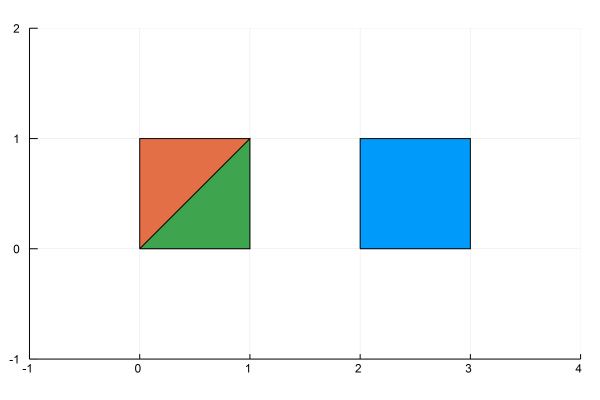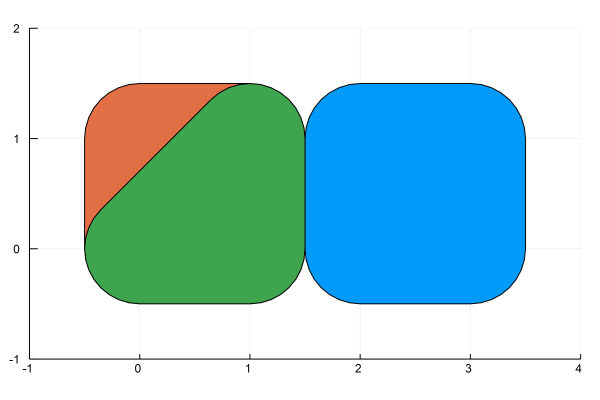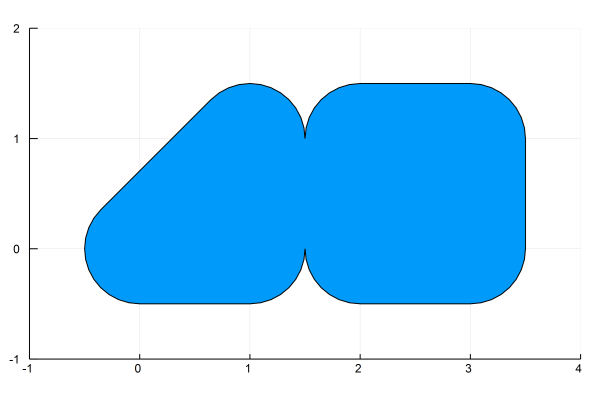dummy-link

32

1

17

13

# LibGEOS.jl

LibGEOS is a package for manipulation and analysis of planar geometric objects, based on the libraries GEOS (the engine of PostGIS) and JTS (from which GEOS is ported). This package wraps the GEOS C API.

Among other things, it allows you to parse Well-known Text (WKT)

``````p1 = readgeom("POLYGON((0 0,1 0,1 1,0 0))")
p2 = readgeom("POLYGON((0 0,1 0,1 1,0 1,0 0))")
p3 = readgeom("POLYGON((2 0,3 0,3 1,2 1,2 0))")
``````Add a buffer around them

``````g1 = buffer(p1, 0.5)
g2 = buffer(p2, 0.5)
g3 = buffer(p3, 0.5)
``````and take the union of different geometries

``````polygon = LibGEOS.union(g1, g3)
``````GEOS functionality is extensive, so coverage is incomplete, but the basic functionality for working with geospatial data is already available. I'm learning as I go along, so documentation is lacking, but if you're interested, you can have a look at the examples in the `examples/` folder, or the tests in `test/test_geo_interface.jl` and `test/test_geos_operations.jl`.

## Installation

1. At the Julia prompt, run

``````pkg> add LibGEOS
``````

This will install both the Julia package and GEOS shared libraries together. The GEOS build comes from GEOS_jll, and the build script can be found in Yggdrasil.

2. Test that `LibGEOS` works by runnning

``````pkg> test LibGEOS
``````

01/30/2015

9 days ago

147 commits